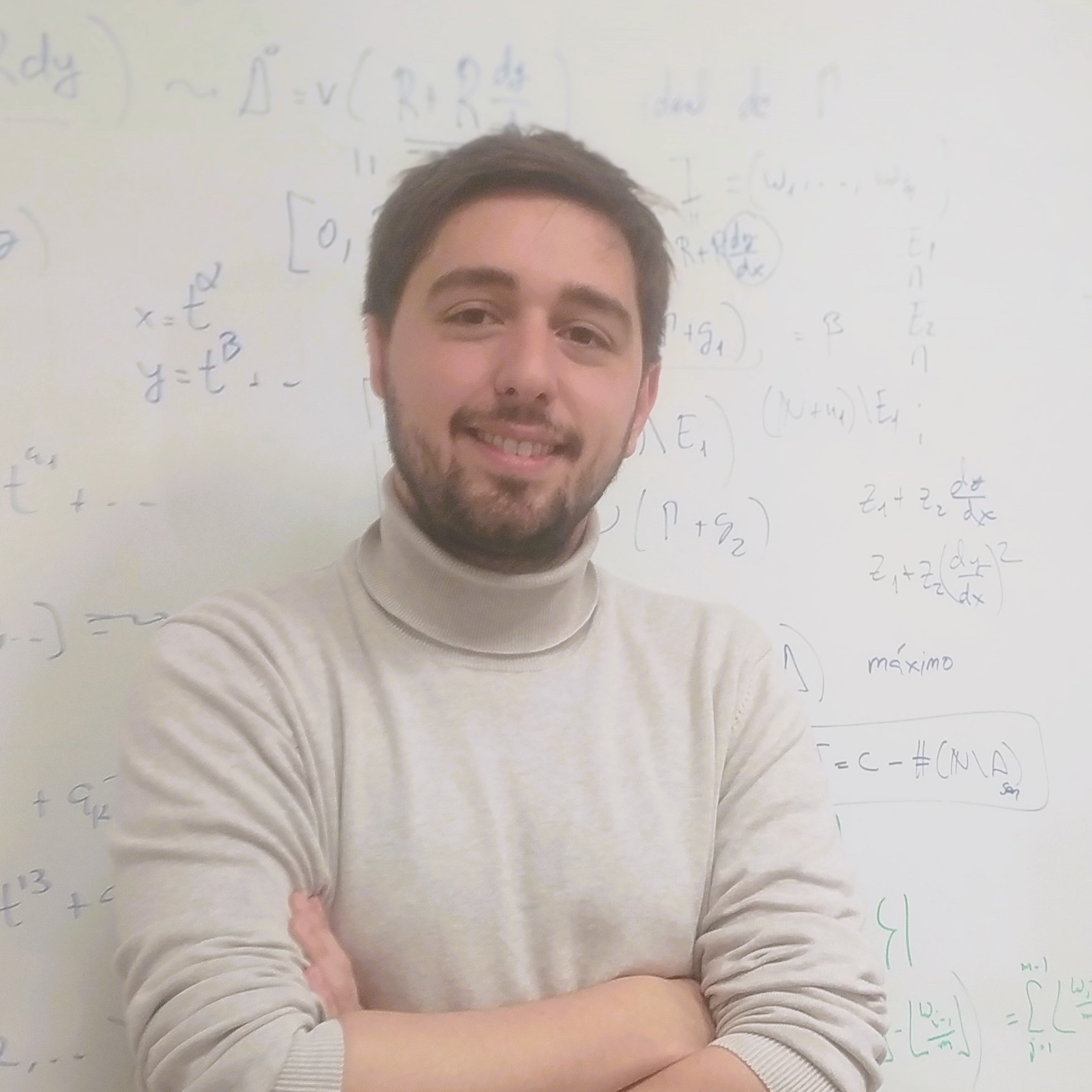# Seminar of Algebra

### The intrinsic topological nature of the Poincaré series of a plane curve singularity

Speaker:
patricioalmiron@ugr.esIn 2003, Campillo, Delgado and Gusein-Zade showed the equality between the Poincaré series of a reducible plane curve singularity $C$ and the Alexander polynomial $\Delta_L$ of the corresponding link $L$. However, their proof lacked of a conceptual explanation for this coincidence. In this talk I will show some new theorems of factorization of the Poincaré series $P_C$ depending on some key values of the semigroup of values of $C$ with purely algebraic methods. As a consequence of these theorems, we will show that our procedure supplies a new proof of the theorem of Campillo, Delgado and Gusein-Zade. More concretely, we will focus on the translation of our algebraic construction to the iterated toric structure of the link $L$. This gives in particular a conceptual reason for the coincidence of $P_C$ and $\Delta_L$.